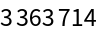#Function Repository Resource:

# GenerateSmoothNumbers

Generate smooth numbers up to some maximum

Contributed by: Sander Huisman
 ResourceFunction["GenerateSmoothNumbers"][max] generates all positive integers up to max whose prime factors are equal to or less than 7. ResourceFunction["GenerateSmoothNumbers"][max,p] generates all positive integers up to max whose prime factors are equal to or less than p.

## Details

ResourceFunction["GenerateSmoothNumbers"][max,p] generates p-smooth numbers.
ResourceFunction["GenerateSmoothNumbers"][max] generates the so-called humble numbers.

## Examples

### Basic Examples (2)

Generate the numbers up to 100 that have factors that are 13 or less:

 In:=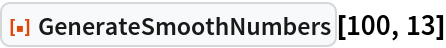Out=Generate the numbers up to 100 that have factors that are 7 or less, so called humble numbers:

 In:=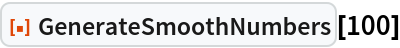Out=### Scope (2)

The second argument does not have to be an integer:

 In:=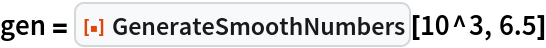Out=Check that the prime factors are all below 6.5:

 In:=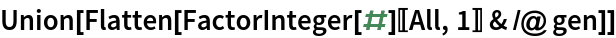Out=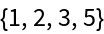### Properties and Relations (2)

Smooth numbers can be found by sieving:

 In:=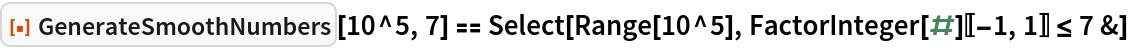Out=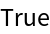The algorithm used by GenerateSmoothNumbers is much faster though:

 In:=Out=### Neat Examples (1)

The argument of GenerateSmoothNumbers can be really large, much larger than practical for a method based on sieving:

 In:=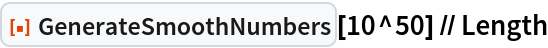Out=